# Welcome back Object Oriented Programming Encapsulation Classes encapsulate

• Slides: 14Welcome back!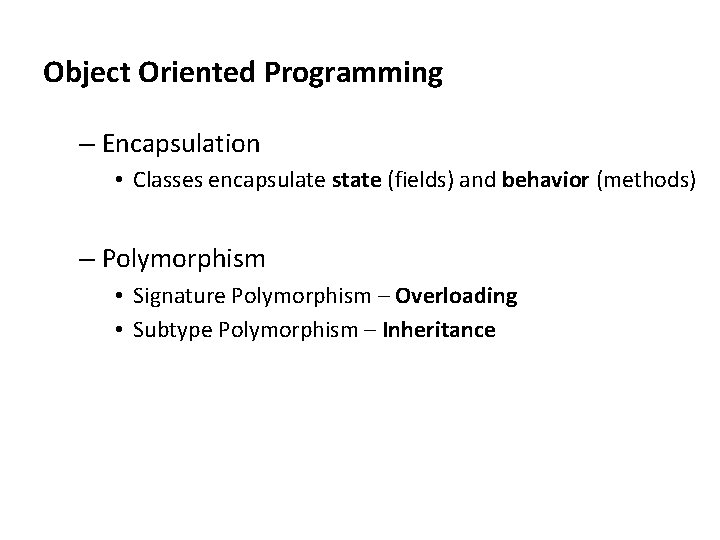Object Oriented Programming – Encapsulation • Classes encapsulate state (fields) and behavior (methods) – Polymorphism • Signature Polymorphism – Overloading • Subtype Polymorphism – InheritanceGets and sets – Instead of accessing data fields directly • ball. x = 5; – Define methods to access them • • • int get. X () { return x; } int get. Foo () { return foo; } void set. X(int x) {this. x = x; } void set. Foo(int foo) {this. foo = foo; } ball. set. X(5);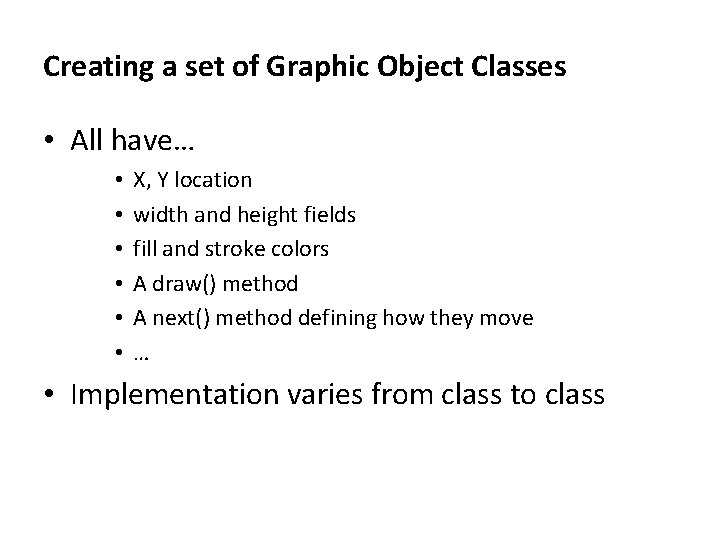Creating a set of Graphic Object Classes • All have… • • • X, Y location width and height fields fill and stroke colors A draw() method A next() method defining how they move … • Implementation varies from class to class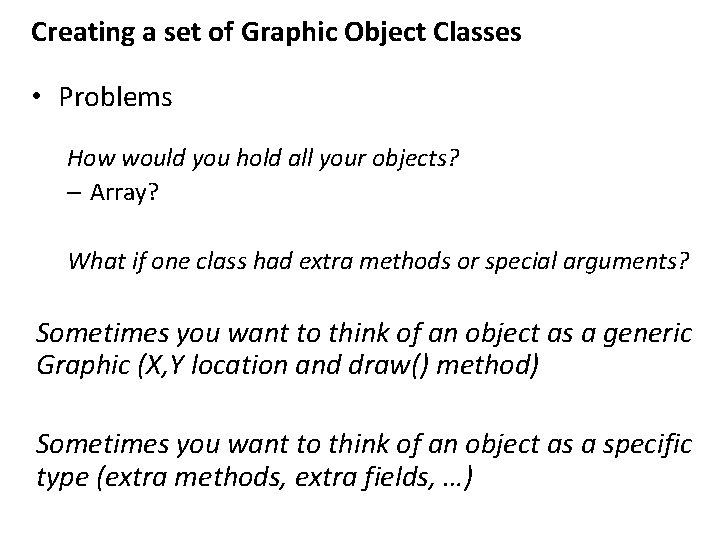Creating a set of Graphic Object Classes • Problems How would you hold all your objects? – Array? What if one class had extra methods or special arguments? Sometimes you want to think of an object as a generic Graphic (X, Y location and draw() method) Sometimes you want to think of an object as a specific type (extra methods, extra fields, …)Rectangle Ellipse Square Circle Graphic X, Y fields draw() method … Arc Curve Shape diameter Inheritance gives you a way to relate your objects in a hierarchical manner More Specific More General Graphic Object Hierarchy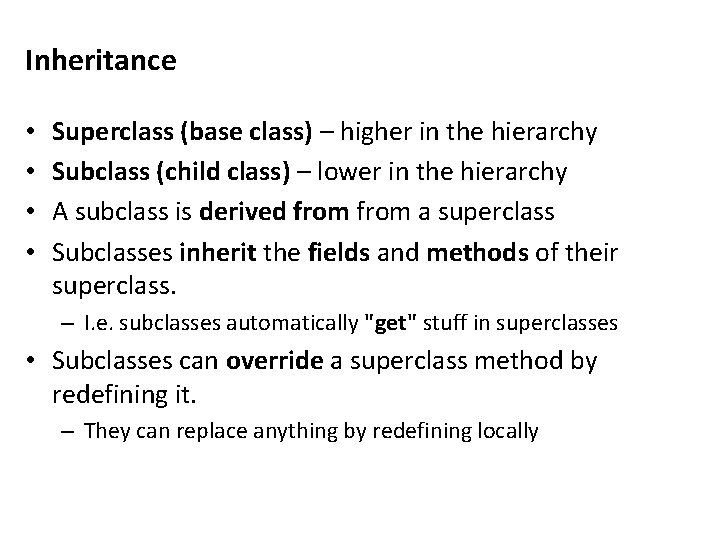Inheritance • • Superclass (base class) – higher in the hierarchy Subclass (child class) – lower in the hierarchy A subclass is derived from a superclass Subclasses inherit the fields and methods of their superclass. – I. e. subclasses automatically "get" stuff in superclasses • Subclasses can override a superclass method by redefining it. – They can replace anything by redefining locally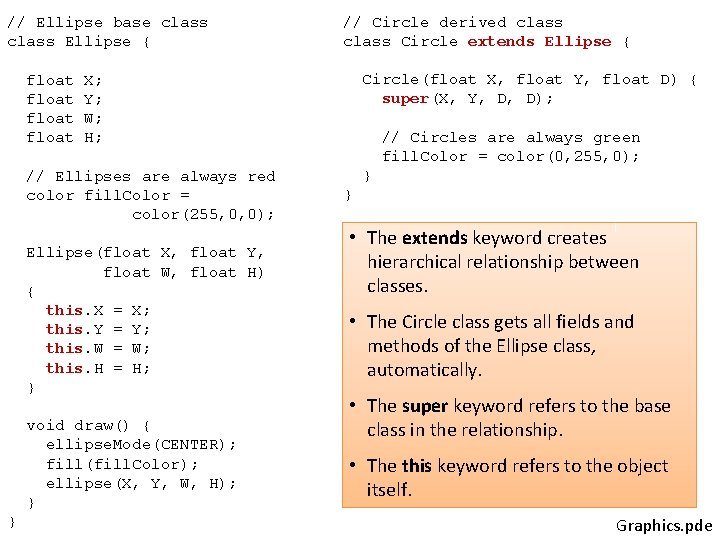// Ellipse base class Ellipse { float Circle(float X, float Y, float D) { super(X, Y, D, D); X; Y; W; H; // Ellipses are always red color fill. Color = color(255, 0, 0); Ellipse(float X, float Y, float W, float H) { this. X = X; this. Y = Y; this. W = W; this. H = H; } void draw() { ellipse. Mode(CENTER); fill(fill. Color); ellipse(X, Y, W, H); } } // Circle derived class Circle extends Ellipse { // Circles are always green fill. Color = color(0, 255, 0); } } • The extends keyword creates hierarchical relationship between classes. • The Circle class gets all fields and methods of the Ellipse class, automatically. • The super keyword refers to the base class in the relationship. • The this keyword refers to the object itself. Graphics. pde// Graphics Ellipse e = new Ellipse(150, 250, 150, 50); Circle c = new Circle(350, 250, 75); void setup() { size(500, 500); smooth(); } void draw() { e. draw(); c. draw(); } Graphics. pde// Graphics 2 Ellipse[] e = new Ellipse; void setup() { size(500, 500); smooth(); for (int i=0; i<e. length; i++) { float X Y W H = = random(0, width); random(0, height); random(10, 100); // Ellipses are Circles are // stored in the same array if (random(1. 0) < 0. 5) e[i] = new Ellipse(X, Y, W, H); else e[i] = new Circle(X, Y, W); } } void draw() { for (int i=0; i<e. length; i++) e[i]. draw(); } Ellipses and Circles in the same array! Graphics 2. pde// Ellipse base class Ellipse { float // Circle derived class Circle extends Ellipse { Circle(float X, float Y, float D) { super(X, Y, D, D); X; Y; W; H; // Circles are always green fill. Color = color(0, 255, 0); } // Ellipses are always red color fill. Color = color(255, 0, 0); Ellipse(float X, float Y, float W, float H) { this. X = X; this. Y = Y; this. W = W; this. H = H; } void draw() { ellipse. Mode(CENTER); fill(fill. Color); ellipse(X, Y, W, H); } // Change color of circle when clicked void mouse. Pressed() { if (dist(mouse. X, mouse. Y, X, Y) < 0. 5*W) fill. Color = color(0, 0, 255); } } • The mouse. Pressed behavior of the Circle class overrides the default behavior of the Ellipse class. // Do nothing void mouse. Pressed() {} } Graphics 3. pde// Graphics 3 Ellipse[] e = new Ellipse; void setup() { size(500, 500); smooth(); // Stuff removed … } void draw() { for (int i=0; i<e. length; i++) e[i]. draw(); } void mouse. Pressed() { for (int i=0; i<e. length; i++) e[i]. mouse. Pressed(); } Graphics 3. pdeA few more rules about inheritance … • A child’s constructor is responsible for calling the parent’s constructor • The first line of a child’s constructor should use the super reference to call the parent’s constructor • The super reference can also be used to reference other variables and methods defined in the parent’s class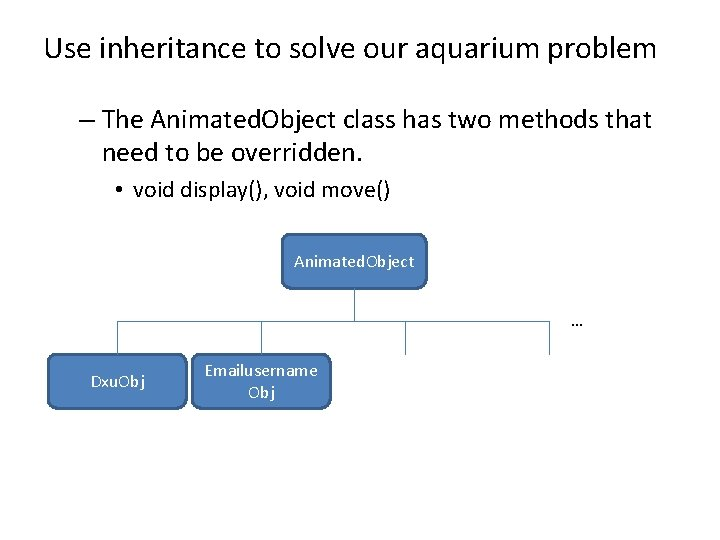Use inheritance to solve our aquarium problem – The Animated. Object class has two methods that need to be overridden. • void display(), void move() Animated. Object … Dxu. Obj Emailusername Obj